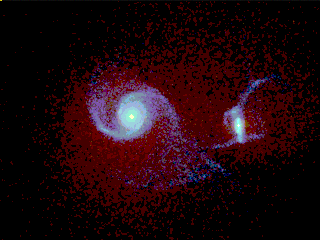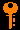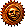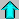# Lecture 23 - Expansion of the Universe(4/13/99)Galaxies --- | --- Large Scale StructureReading:
Chapter 22 (ZG4)

Notes:
pages 93-96

Simulation of a collision between two spiral galaxies. (Courtesy Joshua Barnes/IFA Hawaii)
Key Question:## Investigations:

1. Extragalactic Distance Indicators
• How are the fundamental galaxy parameters R, L, M and v related? What are the observables?
• Why is knowing the distance to things so important?
• What do we mean by standard candles and standard rods?
• If M_V ~ -6 for brightest Cepheids, how far away can they be seen if our apparent magnitude limit is V < -29?
• If the brightest type I and II supernovae have M_V ~ -20, how far can they be seen?
• What are the relative rarities of Cepheids and SN?
• Why are Cepheids useful nearby while SN are best at greater distances?
• What do we mean by the distance ladder, and what are the main steps in bootstrapping the distance scale?
• Why is the surface brightness I = f/W independent of distance, and why does it have units of Lsun/pc^2?
• What do we mean by a surface brightness in magnitudes per square arcsecond? What is the night sky brightness in B-mag/arcsec^2?
• What assumptions do we need to make to derive

L ~ v4

from our fundamental parameters?
• What is the Tully-Fisher relation for spirals?
• What is the Faber-Jackson relation for ellipticals?
• What is the typical central surface brightness for the disk of a spiral galaxy in B-mag/arcsec^2?
2. The Expansion of the Universe
• What did V.M. Slipher find when he measured Doppler shifts for some 40 spiral nebulae?
• What did Edwin Hubble discover when he measured Cepheids in Slipher's galaxies?
• How can the galaxies be receding in all directions from us without us being the center of the Universe?
• What are the implications of the Hubble relation

v = H d

and why does this lead us to the idea of an expanding universe?
• What is the significance of the Hubble constant H, and why are its units km/s/Mpc?
• Why has Hubble's original determination H ~ 500 km/s/Mpc been converging in the latest measurements to H ~ 60 km/s/Mpc? Has the Universe changed?
• How can the Hubble relation be used as a distance indicator, and what problems might arise?

## Expansion of the Universe in Outline

1. Distance Indicators
• Distances are quoted in megaparsecs, Mpc, or 10^6 pc.
• Variable Stars - Cepheids and RR Lyrae stars
• Supernovae Type I and II
• Planetary nebulae
• H II regions
• Brightest O and B stars
• Surface brightness fluctuations
• Spiral rotation velocities
• Elliptical velocity dispersions
• Globular cluster sizes and/or brightness
• Galaxy luminosities or diameters
• The Hubble distance-redshift relation
2. The Hubble Relation
• Edwin Hubble (1929) discovers galaxies moving away from us -> expanding universe!
• Velocity from Doppler shift (redshift = moving away)
• speed proportional to distance = Hubble relation: v = H d
• Hubble constant H = 50 - 100 km/s per Mpc of distance
• Current best value (HST): H = 82 km/s/Mpc
• Relation v = H d corresponds to uniform expansion of Universe
• Not due to actual motion of galaxies away from us (no explosion)
• Redshift z (approx. v/c at small v) is a measure of the distance
• At small redshifts (z << 1): d = cz/H
• Most distant galaxies known: z > 3 ( d > 3600 Mpc )!
• In addition to a Hubble velocity Hd, a galaxy may have a peculiar velocity vpec which is a real motion in space.
• For example, our own Milky Way galaxy has a peculiar velocity of 540 km/s!

## Finding Distances to Galaxies

One of the most crucial measurements in astronomy is that of finding the distance to a particular object, be it a planet, star, galaxy, quasar, or cluster of galaxies. The use of Cepheid variable stars for distances in the galaxy was discussed in Lecture 20. As Cepheids have an absolute magnitude of M_v ~ -6 or fainter, these are good in our galaxy, and to relatively nearby galaxies (100 Mpc at most). We need a distance ladder to take us out into the far extragalactic reaches of space.

Relations between the size, luminosity, mass, and internal velocities provide us with some of our most useful distance indicators. Known as the Tully-Fisher relation for spirals, and the Faber-Jackson relation for ellipticals, these empirical relations between the observed Doppler line widths and the true luminosity of a galaxy allow us to use it as a standard candle.

For an explanation of these relations, see the following resources:

In summary:

To determine the physical properties of galaxies, we first need to find the distance to any given galaxy we wish to study. We use distance indicators to find the distance. Galaxies are so far away that there is no hope of using a direct method such as parallax. We must resort to other distance indicators, many of which are approximate. To quote distances to galaxies, we use the unit megaparsec, Mpc, which is 10^6 pc.

The primary distance indicators for nearby galaxies are variable stars such as Cepheids and RR Lyrae stars. The period-luminosity relation for these stars can be calibrated from observations of variable stars in our own galaxy. Unfortunately, these stars are relatively faint, with absolute visual magnitudes of -6 or fainter. Thus, with a powerful telescope with a limit of apparent magnitude 24, we can see out to

5 log d = m - M + 5 = 24 - (-6) + 5 = 35

or d = 10 Mpc. In addition, for distant galaxies the variable stars are lost in the myriad of other bright stars. One of the main goals of the Hubble Space Telescope was to measure Cepheid variables in galaxies out to the Virgo cluster (see below) at a distance of around 20 Mpc. The results from this study have been published this last year, and give us our best measurements to galaxies this far away.

Supernovae are also used as distance indicators. These can be seen much further away since they have absolute magnitudes from -16 to -20. Both Type I supernovae and the fainter Type II have been used. There is a general relation between the time it takes a supernova to fade a given number of magnitudes and its intrinsic luminosity, which makes these objects useful as distance indicators.

Other objects or approximately known intrinsic brightness are also used, and the distance deduced from the distance modulus. These indicators are called standard candles. Examples of bright objects used as standard candles are planetary nebulae, H II regions, the brightest O and B stars in galaxies, and surface brightness fluctuations in galaxies caused by the brightest stars, and the brightness of globular clusters. These have not very high accuracies because there is no good way to get the intrinsic luminosities of these things, and they come in a range of luminosities.

Other methods which sort of involve standard candles are the relationships between the maximum rotation velocity of a spiral galaxy disk and the luminosity of the galaxy (the Tully-Fisher relation) and between the central velocity dispersion and the central surface brightness of an elliptical galaxy (the Faber-Jackson relation). When calibrated on nearby galaxies with Cepheid distances, these two relations are the main distance indicators for galaxies from 10 to 100 Mpc away.

The intrinsic luminosities of galaxies themselves make poor standard candles, but when there are many galaxies in a group or cluster all at the same distance, then the brightest galaxies do have approximately the same luminosity. This is a distance indicator of the last resort, due to its large error bar.

Object of a known size can be used as distance indicators through the small angle formula for the apparent angular size versus distance. These are called standard yardsticks (I guess metersticks would be more appropriate). Examples of these are the angular diameters of globular clusters or galaxies.

The final distance indicator is the Doppler velocity of a distant galaxy. This discovery by Hubble in 1929 really set the stage for cosmology.Prev Lecture ---Next Lecture ---Astr12 Index ---Astr12 Home

smyers@nrao.edu Steven T. Myers